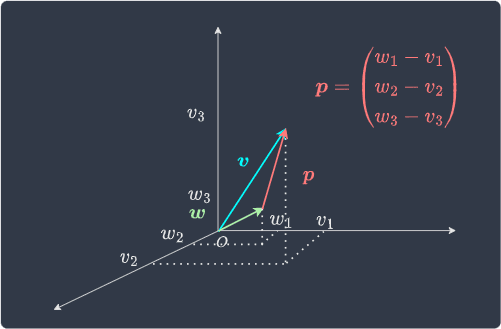search
Search
Map of Data Science
Guest 0reps
exit_to_appLog out
Map of data science
Thanks for the thanks!
close
Cancel
Post
account_circle
Profile
exit_to_app
Sign out
search
keyboard_voice
close
Searching Tips
Search for a recipe:
"Creating a table in MySQL"
Search for an API documentation: "@append"
Search for code: "!dataframe"
Apply a tag filter: "#python"
Useful Shortcuts
/ to open search panel
Esc to close search panel
to navigate between search results
d to clear all current filters
Enter to expand content previewDoc SearchCode Search BetaSORRY NOTHING FOUND!
mic
Start speaking...Voice search is only supported in Safari and Chrome.
Shrink
Navigate to
A
A
brightness_medium
share
arrow_backShareTwitterFacebook
check_circle
Mark as learned
thumb_up
1
thumb_down
0
chat_bubble_outline
1
auto_stories new
settings

# Guide on Position Vectors in Linear Algebra

Linear Algebra
chevron_right
Vectors
schedule Jan 8, 2023
Last updated
local_offer Linear Algebra
Tags
expand_more
map
Check out the interactive map of data science

# What are position vectors?

Recall that vectors can be represented as arrows that connect one point to another. Two vectors are considered to be equal as long as their magnitude and direction are the same. For instance, consider the following two vectors: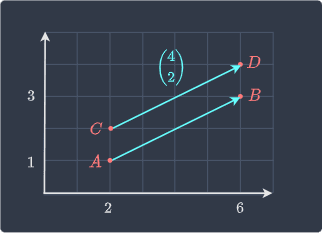These two vectors are equal even though they have different starting and ending points. In this sense, these types of vectors are described as "free" because we care only about their magnitude and direction and not about their specific starting and ending point.

In contrast, the so-called position vectors always have the tail at the origin. For instance, the following vector is a position vector: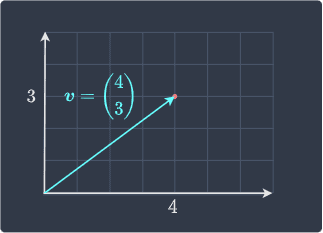Because position vectors begin at the origin, we can easily express any point on the coordinate system. For example, the above vector represents the point $(4,3)$. As you would expect, the point $(5,2)$, for instance, will be represented by the following vector: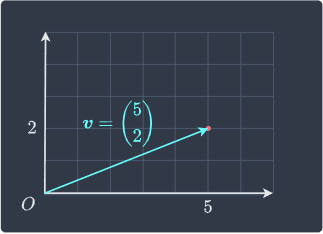Unlike free vectors, position vectors are unique given a magnitude and a direction.

Theorem.

# Vector between two points

Suppose we have two points $A$ and $B$ and we wish to find the vector $\overrightarrow{AB}$ shown below: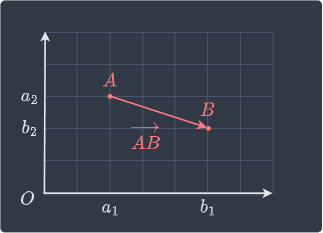The vector $\overrightarrow{AB}$ is computed by:

$$\overrightarrow{AB}= \begin{pmatrix} b_1-a_1\\b_2-a_2 \end{pmatrix}$$

Proof. Suppose we have two points $A$ and $B$ and we wish to find the vector $\overrightarrow{AB}$ shown below:One approach to find $\overrightarrow{AB}$ is to use vectors $\overrightarrow{OA}$ and $\overrightarrow{OB}$ below: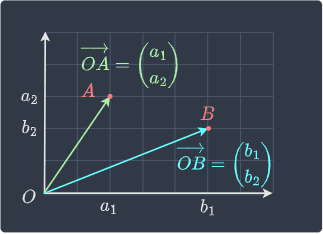Recall the head-to-tail methodlink of vector addition - vector $\overrightarrow{AB}$ can be computed as follows:

\begin{align*} \overrightarrow{AB}&= -\overrightarrow{OA}+\overrightarrow{OB}\\ &= \overrightarrow{OB}-\overrightarrow{OA}\\ &= \begin{pmatrix} b_1-a_1\\b_2-a_2 \end{pmatrix} \end{align*}

Visually, we are reversing the direction of $\overrightarrow{OA}$ by multiplying it by $-1$ so that we can find $\overrightarrow{AB}$ like so: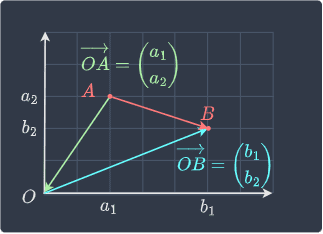This completes the proof.

Theorem.

## Computing the vector between two points

Find vector $\overrightarrow{AB}$ below: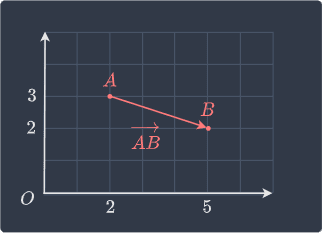Solution. Let's directly use the theorem:

\begin{align*} \overrightarrow{AB} &= \begin{pmatrix} b_1-a_1\\b_2-a_2 \end{pmatrix}\\ &= \begin{pmatrix} 5-2\\2-3 \end{pmatrix}\\ &= \begin{pmatrix} 3\\-1 \end{pmatrix} \end{align*}

We can see that this is true in the diagram as well.

# Position vectors in higher dimensions

We have only discussed position vectors in two-dimensional space but they can reside in any dimension. For instance, a position vector in $\mathbb{R}^3$ is shown below: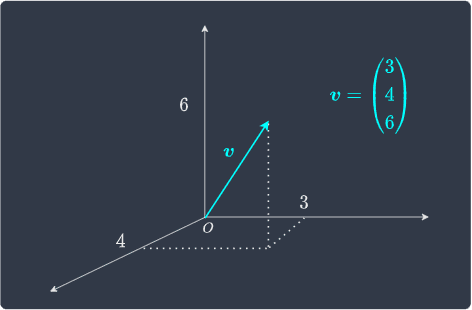The vector between two points in $\mathbb{R}^3$ is: